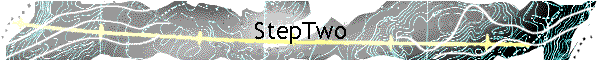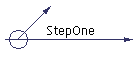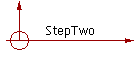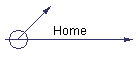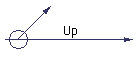Result of the count:

 a b c d e f g h I j k l m n o p q r s t u v w x y z 3 5 0 3 1 17 2 5 8 21 7 5 15 13 0 0 8 7 8 19 5 0 9 11 17 1

Order of frequency of occurrence in the encrypted tablet:  j (21), t (19), f (17) , m (15)

Now, again using English since most of us read neither Aramaic nor Greek, an analysis of the English language indicates the letter "e" occurs most frequently, followed by "t, n, o, and i"  in descending order.

Substituting "e" for the most frequent letter in the worksheet "j" and so on.

Now try this substitution to test the theory: ("e" for "j" indicated by the blue letters)

 y m j n r f l j t k h m w n x y t h e e q n j x g j m n i y m j l w j j s e e e e b f y j w y m j b f d y t y m j e q n e m y y m j b f d y t e j a j u g f x y n s l e e h m w n x y x t r t k l t i f s I k f y m j w x f a n t w e t k h f j x f w u m n q n u u n e n s y m j x m f I t b t k y m j r t z s y t k e r t x j x q t a j t k l t i e e r f s b t r f s f s i h m n q i f w j u w t h q f d r j i y t f q q e e

Look at the "e" patterns - many three letter words - now we step to the "common word" approach.

Substitute "t" and "h" in the first word to get "the."

With just this limited amount of information, set up a simple "monoalphabetic shift pattern," where the alphabet is lined up in traditional sequence, but with the start point offset - see where the "a" in the two rows are offset.

The top line is the code and the bottom line is the plain text result of de-coding. It appears the sample word "the" fits perfectly.

 j k l m n o p q r s t u v w x y z a b c d e f g h I e f g h I j k l m n o p q r s t u v w x y z a b c d